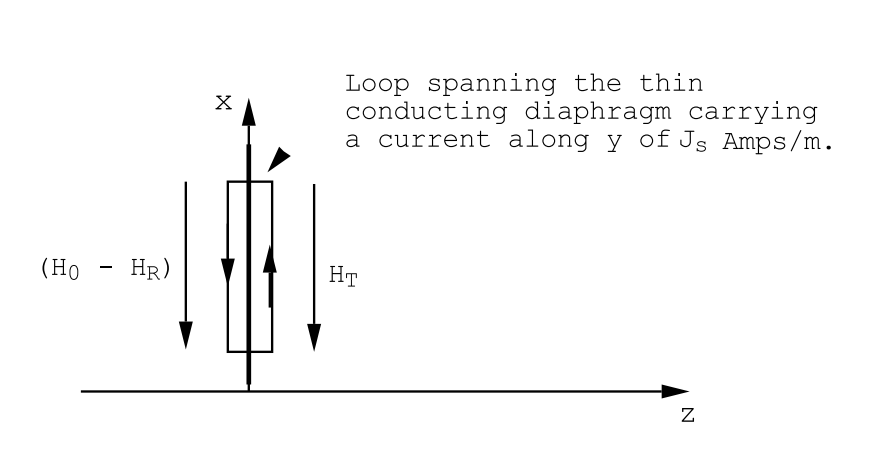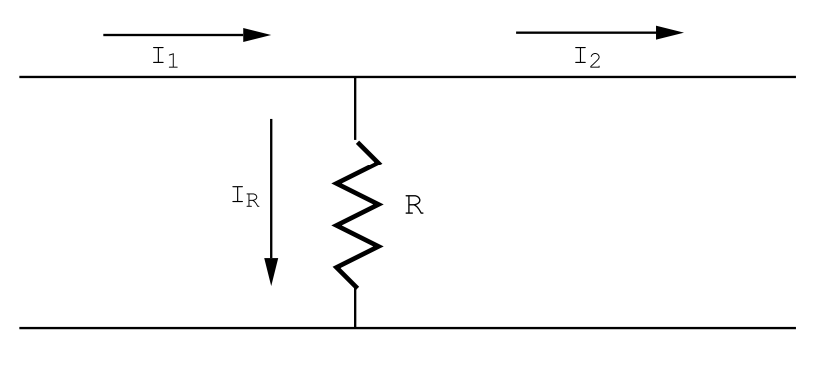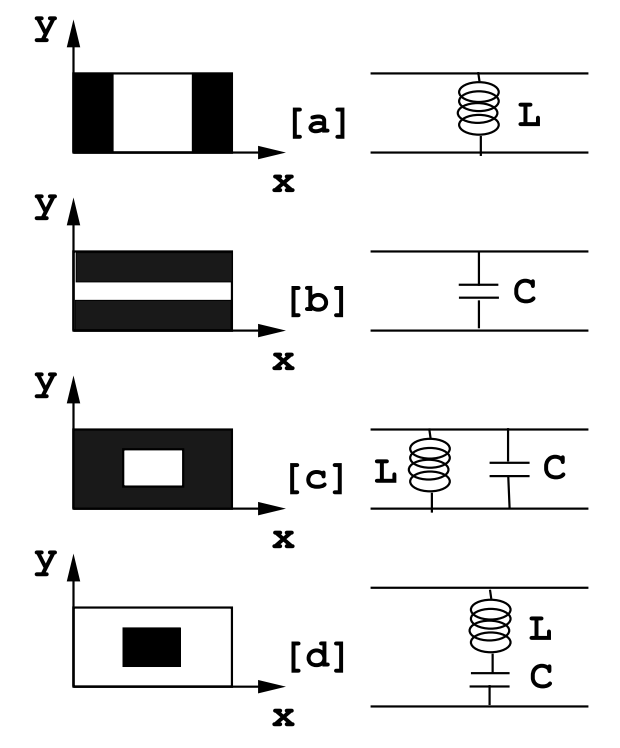$$\require{cancel}$$

# 12.3: Waveguide Discontinuities

Any discontinuity in the dielectric constant, in the permeability, or any discontinuity in the dimensions of a waveguide will result in reflected waves. For simplicity, we will discuss the case in which the waveguide will support the propagation of only the TE10 mode. A forward propagating TE10 mode that encounters an obstacle in the waveguide will, in general, be partially reflected and partially transmitted. This can be illustrated for a particularly simple obstacle; let a very thin sheet of material of thickness $$d$$ and characterized by a conductivity σ be placed across the guide as illustrated in Figure (12.3.5).Figure $$\PageIndex{5}$$: A TE10 wave incident on a conducting diaphragm placed across the waveguide.Figure $$\PageIndex{6}$$: A TE10 wave incident on a conducting diaphragm placed across the waveguide. The magnetic fields on each side of the diaphragm are related by the condition curl($$\vec H$$) = Js .

The thickness of the sheet, d, is assumed to be very thin compared with the skindepth $$\delta=\sqrt{2 /\left(\omega \sigma \mu_{0}\right)}$$, see Chpt.(10), Section(10.4). The thickness d is also assumed to be very small compared with the wavelength λg = 2$$\pi$$/kg. The tangential component of the electric field in this case, Ey, must be continuous across the diaphragm

$\mathrm{E}_{\mathrm{T}}=\mathrm{E}_{0}+\mathrm{E}_{\mathrm{R}}. \label{12.40}$

The electric field at the conducting diaphragm generates a current density along y whose magnitude is Jy = σET. When integrated across the film thickness d this current density produces an equivalent surface current sheet having the strength

$\mathrm{J}_{\mathrm{s}}=\sigma d \mathrm{E}_{\mathrm{T}} \quad \text { Amps } / m. \nonumber$

This current sheet causes a discontinuity in the tangential component of $$\vec H$$ from Amp`ere’s law; i.e. the magnetic field components, Hx, on either side of the film are related by

$\int \int_{L o o p} \operatorname{curl}(\overrightarrow{\mathrm{H}}) \cdot \mathrm{d} \overrightarrow{\mathrm{S}}=\oint_{C} \overrightarrow{\mathrm{H}} \cdot \mathrm{d} \overrightarrow{\mathrm{L}}=\operatorname{total} \text { current through the Loop.} \label{12.41}$

The loop in question is illustrated in Figure (12.3.6). Condition (\ref{12.41}) results in the equation

$\mathrm{H}_{0}-\mathrm{H}_{\mathrm{R}}-\mathrm{H}_{\mathrm{T}}=\mathrm{J}_{\mathrm{s}}=\sigma d \mathrm{E}_{\mathrm{T}}, \nonumber$

or using the waveguide impedance, ZG = ωµ0/kg,

$\frac{\left(\mathrm{E}_{0}-\mathrm{E}_{\mathrm{R}}-\mathrm{E}_{\mathrm{T}}\right)}{\mathrm{Z}_{\mathrm{G}}}=\sigma d \mathrm{E}_{\mathrm{T}}, \nonumber$

or

$\mathrm{E}_{0}-\mathrm{E}_{\mathrm{R}}=\left[1+\mathrm{Z}_{\mathrm{G}} \sigma d\right] \mathrm{E}_{\mathrm{T}}. \label{12.42}$

Equations (\ref{12.40}) and (\ref{12.42}) can be solved to obtain the reflected and transmitted wave amplitudes:

\begin{align} \mathrm{R} &=\frac{\mathrm{E}_{\mathrm{R}}}{\mathrm{E}_{0}}=-\frac{\mathrm{Z}_{\mathrm{G}} \sigma \mathrm{d}}{\left(2+\mathrm{Z}_{\mathrm{G}} \sigma \mathrm{d}\right)}, \label{12.43} \\ \mathrm{T} &=\frac{\mathrm{E}_{\mathrm{T}}}{\mathrm{E}_{0}}=\frac{2}{\left(2+\mathrm{Z}_{\mathrm{G}} \sigma \mathrm{d}\right)}. \nonumber \end{align}Figure $$\PageIndex{7}$$: A transmission line, characteristic impedance Z0 Ohms, shunted by a resistance of R Ohms. The incident wave amplitude is V0 Volts; the reflected wave amplitude is VR Volts; the transmitted wave amplitude is VT Volts. The reflection coefficient is R = VR/V0 = −(Z0/R)/ [2 + (Z0/R)]. The transmission coefficient is T = VT/V0 = 2/ [2 + (Z0/R)].

These reflection and transmission coefficients are the same as would be calculated for the problem of a resistor, R=1/σd, placed across a transmission line whose characteristic impedance is ZG, Figure (12.3.7). The close analogy between waveguide problems and transmission line problems has been stressed in the article by H.G.Booker,”The Elements of Wave Propagation using the Impedance Concept”, Electrical Engineering Journal, Volume 94,pages 171- 198, 1947. The analogy holds for more general impedances. For an incident TE10 mode a thin wire placed across the waveguide parallel with the electric field has the same reflection and transmission coefficients as an inductive reactance placed across a transmission line, Figure (12.3.8). Similarly, the thin metal diaphragms illustrated in Figure (12.3.9) give rise to reflection and transmission coefficients for the waveguide that are the same as would be produced by the circuits on the right hand side of Figure (12.3.9) if they were to be placed across a transmission line.

A thin metal diaphragm containing a hole such as that shown in Figure (12.3.9c) can be used to produce a resonant cavity, Figure (12.3.10). The resonant structure is formed by the section of waveguide that is contained between the diaphragm and a waveguide short circuit; the waveguide short simply consists of a metal plate that completely closes off the guide. The hole in the diaphragm need not be rectangular as shown in Figure (12.3.9): it is often convenientFigure $$\PageIndex{8}$$: A transmission line, characteristic impedance Z0 Ohms, shunted by an inductive impedance of ZL Ohms. The incident wave amplitude is V0 Volts; the reflected wave amplitude is VR Volts; the transmitted wave amplitude is VT Volts. The reflection coefficient is R = VR/V0 = i(Z0/Lω)/ [2 − (iZ0/Lω)]. The transmission coefficient is T = VT/V0 = 2/ [2 − (iZ0/Lω)].

to use a round hole. At a frequency such that the length of the cavity, L, is very nearly equal to an integral number of half-wavelengths the reflection from the diaphragm becomes smaller than its value for neighboring frequencies. The reflectivity exhibits a dip when plotted as a function of frequency, Figure (12.3.11). At resonance a standing wave is set up in the cavity that has nodes at the diaphragm and at the shorted end. Energy is fed into the cavity through the coupling hole, and the fields inside the cavity become so large that the energy dissipated in the cavity walls is equal to the energy carried into the cavity through the coupling hole. The Q, or quality factor, for the cavity is defined to be

$\mathrm{Q}=2 \pi\left(\frac{\text { Energy Stored }}{\text { Energy Loss per Cycle }}\right). \label{12.44}$

The quality factor for a microwave cavity commonly exceeds 1000 and is often found to be as large as 10,000. The electric and magnetic fields in a properly coupled microwave cavity range in amplitude between 30 and 100 times as large as the amplitudes of the incident wave that feeds the cavity. At resonance the cavity appears to be a pure resistance. The valueFigure $$\PageIndex{9}$$: Thin, perfectly conducting metal diaphragms placed across a waveguide and their equivalent shunt circuits placed across a transmission line. Shaded areas are metal, unshaded areas are unobstructed.Figure $$\PageIndex{10}$$: A microwave cavity resonator. Resonance occurs when L = nλg/2 where λg = 2$$\pi$$/kg and n is an integer.Figure $$\PageIndex{11}$$: The absolute value of the reflection coefficient as a function of frequency for a microwave signal having an amplitude of E0 Volts/m incident on a microwave cavity. The reflected wave amplitude is ER Volts/m. The quality factor of the cavity is given by Q=f0/∆f where ∆f is the frequency interval between the frequencies for which the change in reflectivity is 0.7 of the maximum change.

of that resistance depends upon the size of the coupling aperture. For a particular orifice size, a size that depends upon the cavity losses and which must be determined by trial and error, the cavity absorbs all of the energy that is incident upon it; the cavity forms a matched load. Under matched conditions the reflectivity of the cavity is very sensitive to changes in the cavity losses, and this configuration is often used to measure the magnetic field dependence of the absorption of microwave energy by electron spins (electron spin resonance absorption or ESR).

The fields in the microwave cavity form standing waves at resonance. For a TE10 mode

\begin{align} \mathrm{E}_{\mathrm{y}}=\mathrm{E}_{0} \sin \left(\frac{\pi \mathrm{x}}{\mathrm{a}}\right) \sin \left(\mathrm{k}_{\mathrm{g} \mathrm{z}}\right) \exp (-i \omega \mathrm{t}), \label{12.45} \\ \mathrm{H}_{\mathrm{x}}=+i\left(\frac{\mathrm{k}_{\mathrm{g}}}{\omega \mu_{0}}\right) \mathrm{E}_{0} \sin \left(\frac{\pi \mathrm{x}}{\mathrm{a}}\right) \cos \left(\mathrm{k}_{\mathrm{g}} \mathrm{z}\right) \exp (-i \omega \mathrm{t}), \nonumber\\ \mathrm{H}_{\mathrm{z}}=-i\left(\frac{\pi}{\omega \mu_{0} \mathrm{a}}\right) \mathrm{E}_{0} \cos \left(\frac{\pi \mathrm{x}}{\mathrm{a}}\right) \sin \left(\mathrm{k}_{\mathrm{g}} \mathrm{z}\right) \exp (-i \omega \mathrm{t}), \nonumber \end{align}

The zero for z in Figure (12.3.10) is located at the diaphragm. The length of the cavity must be chosen so that kgL = m$$\pi$$, where m is an integer, so that the electric field vanishes on the cavity end walls. Notice that the electric and magnetic fields are 90 out of phase. This means that the energy stored in the cavity swings forth and back between energy stored in the electric field and energy stored in the magnetic field: the total energy is independent of the time. For this cavity resonator, and for m odd, the electric field is large in the central region, i.e. at x=a/2, z=L/2, and in this region the magnetic fields are small. Conversely, the magnetic fields are large at the cavity walls where the electric field is small. The energy losses due to eddy currents flowing in the cavity walls can be estimated using the considerations described in the next section.